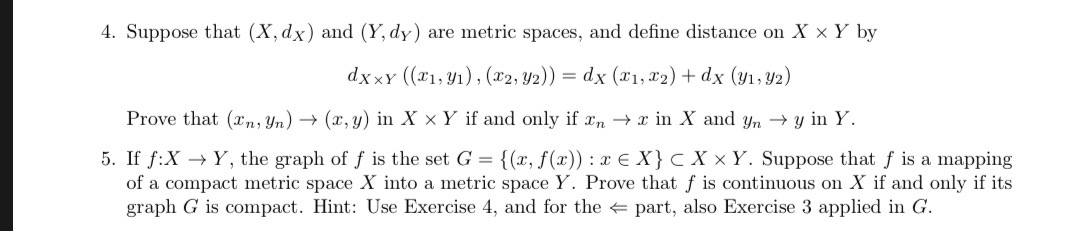4. Suppose that (X, dx) and (Y, dy) are metric spaces, and define distance on X × Y byProve that (Tmyn) → (z, y) in X × Y if and only ifFm → z in X and Un → y in Y.5. If f:X → Y, the graph of f is the set G = {(x, f(x)) : x E X}X × Y. Suppose that f is a mappingof a compact metric space X into a metric space Y. Prove that f is continuous on X if and only if itsgraph G is compact. Hint: Use Exercise 4, and for the - part, also Exercise 3 applied in G.

Questionhelp_outlineImage Transcriptionclose4. Suppose that (X, dx) and (Y, dy) are metric spaces, and define distance on X × Y by Prove that (Tmyn) → (z, y) in X × Y if and only ifFm → z in X and Un → y in Y. 5. If f:X → Y, the graph of f is the set G = {(x, f(x)) : x E X} X × Y. Suppose that f is a mapping of a compact metric space X into a metric space Y. Prove that f is continuous on X if and only if its graph G is compact. Hint: Use Exercise 4, and for the - part, also Exercise 3 applied in G. fullscreen
Step 1

Hey, since there are multiple questions posted, we will answer first question. If you want any specific question to be answered then please submit that question only or specify the question number in your message.

Step 2

Problem 4:

Given that (X, dX) and (Y, dY) are the metric spaces and the distance is defined as follows.

dX x Y ((x1 , y1), (x2 , y2)) = dX  (x1 , x2) + dY (y1 , y2)

Step 3

To prove that (xn , yn) tends to (x, y) in X x Y if and only if xn tends to x inand yn t...

Want to see the full answer?

See Solution

Want to see this answer and more?

Our solutions are written by experts, many with advanced degrees, and available 24/7

See Solution
Tagged in

Math